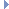﻿ MGeometryManager Class Reference

# MGeometryManager Class Reference

MGeometryManager Class ReferenceRelated help topics:

`#include <MGeometryManager.h>`

## Class Description

This method is obsolete.

Deprecated:

This class provides methods for managing MGeometry resources.

## Public Types

enum  GeometricShape { kDefaultSphere = 0, kDefaultPlane, kDefaultCube }
Default geometry shapes. More...

## Static Public Member Functions

static MGeometry getGeometry (const MDagPath &shape, const MGeometryRequirements &requirements, MObject *components=NULL)
Access the Geometry cache for a shape. More...

static MGeometryListreferenceDefaultGeometry (const MGeometryManager::GeometricShape geomShape, const MGeometryRequirements &requirements)
Obtain a reference to geometry for some "default" shapes maintained by the manager such that the geometry returned will match a set of geometric requirements (MGeometryRequirements). More...

static MStatus dereferenceDefaultGeometry (MGeometryList *data)
This is the companion method to referenceDefaultGeometry() and must always be called immediately after usage of data supplied by the reference call. More...

static const char * className ()
Returns the name of this class. More...

## Member Enumeration Documentation

 enum GeometricShape

Default geometry shapes.

Enumerator
kDefaultSphere

Sphere with radius 1, centered at 0,0,0.

kDefaultPlane

Plane with width and height of 1, centered at 0,0,0.

Assuming "Y-Up" orientation: width = x-axis, and height = y-axis.

kDefaultCube

Cube with width, height and depth of 1, centered at 0,0,0.

## Member Function Documentation

 MGeometry getGeometry ( const MDagPath & shape, const MGeometryRequirements & requirements, MObject * components = `NULL` )
static

Access the Geometry cache for a shape.

Parameters
 [in] shape the surface [in] requirements the surface data you want in the cache. Attempting to access cache data not included in the requirements will fail. [in] components an optional component group, for accessing a sub-selection of faces (e.g. the faces assigned to a material)
Returns
The cached geometry.Examples:
 MGeometryList * referenceDefaultGeometry ( const MGeometryManager::GeometricShape geomShape, const MGeometryRequirements & requirements )
static

Obtain a reference to geometry for some "default" shapes maintained by the manager such that the geometry returned will match a set of geometric requirements (MGeometryRequirements).

The actual form is a geometric iterator (MGeometryList), which can be used to iterate over the internal data kept (MGeometry).

Note that all data is assumed to be "read-only", as the data is not owned by the caller. To maintain proper reference counting of internal data, the user must "deference" the data when no longer using it via the MGeometryManager::dereferenceGeometry() calls. It is recommended to immediately dereference the data after each use. There is negligable overhead to reference and dereference data in this manner.

Available default shapes include:

• A polygonal sphere : Sphere has radius of 1 and is centered at the origin (0,0,0) in object space.
• A polygonal cube : Cube has dimensions of 1 and is centered at the origin (0,0,0) in object space.
• A polygonal plane : Plane has dimensions of 1 is centered at the origin (0,0,0) in object space. Assuming "Y-Up" orientation, the width is the along the x-axis and the height is along the y-axis.

The following is an example usage via a hardware shader plugin for swatch rendering:

{
// Set up a NULL path
MDagPath path;
// Get the requirements from the hardware shader
MGeometryRequirements requirements;
populateRequirements(path, requirements);
// Ask for a default sphere
if(geomIter == NULL) {
}
// Set up where to draw to ....
// Now draw using the geometry iterator returned.
//
for( ; !geomIter->isDone(); geomIter->next())
{
// Get the current geometry
MGeometry& geometry = geomIter->geometry(MGeometryList::kNone);
// Get the current object to world matrix
const MMatrix& mtm = geomIter->objectToWorldMatrix();
glMatrixMode(GL_MODELVIEW);
// Get some data tro draw
MGeometryPrimitive primitives = geometry.primitiveArray(0);
const MGeometryData pos = geometry.position();
const MGeometryData normal = geometry.normal();
if (pos.data() != NULL && primitives.data() != NULL)
{
// Set up position and normals for drawing
//
glEnableClientState(GL_VERTEX_ARRAY);
glVertexPointer(3, GL_FLOAT, 0, (float*) pos.data());
if (normal.data()) {
glEnableClientState(GL_NORMAL_ARRAY);
glNormalPointer(GL_FLOAT, 0, normal.data());
}
// Draw with the current indexing structure
MGeometryPrimitive primitives = geometry.primitiveArray(0);
glDrawElements(GL_TRIANGLES, primitives.elementCount(), GL_UNSIGNED_INT, primitives.data());
glDisableClientState(GL_VERTEX_ARRAY);
if (normal.data())
glDisableClientState(GL_NORMAL_ARRAY);
}
}
// Now dereference the geometry iterator used.
}
Parameters
 [in] geomShape Desired geometry shape. [in] requirements Geometry requirements (MGeometryRequirements).
Returns
• A pointer to a geometry iterator (MGeometryList), which contains a reference to the geometric data (MGeometry). The MGeometry information which tries to match the requirements passed in. ( e.g. position, normal, texture coordinates, tangents, and binormals etc). It can be assumed that all data is floating point such that:

• Positions are 3 float (x,y,z) positions
• Normals are 3 float (x,y,z) vectors.
• Texture coordinates are 2 float (u,v) tuples.
• Tangents are 3 float (x,y,z) vectors.
• Binormals are 3 float (x,y,z) vectors.Examples:
 MStatus dereferenceDefaultGeometry ( MGeometryList * geomIterator )
static

This is the companion method to referenceDefaultGeometry() and must always be called immediately after usage of data supplied by the reference call.

This call simply maintains proper internal state for any data used.

Parameters
 [in] geomIterator The geometry iterator returned from referenceDefaultGeometry().
Returns
MStatus::kSuccess if successfull, otherwise MStatus::kFailure.Examples:
 const char * className ( )
static

Returns the name of this class.

Returns
The name of this class.

The documentation for this class was generated from the following files:
• MGeometryManager.h
• MGeometryManager.cpp